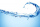# Mixed numbers + subtraction - math problems

#### Number of problems found: 6

• BenhurBenhur boiled 1 1/4 liters of water in a kettle. After 10 1/2 minutes he measured the water again. It had 3/4 liters left in the kettle. What is the amount of water that evaporates every minutes?
• Jose studiedJose studied for 4 and 1/2 hours on Saturday and another 6 and 1/4 hours on Sunday. How many subjects did he study if he has alloted 1 and 1/2 hours per subject on Saturday and 1 and 1/4 hours per subject on Sunday?
• SubtractSubtract two mixed numbers: 3 1/2 -1 3/4
• Sum of 20Sum of the two numbers is 15 1/6. If one of the numbers is 2 2/3, find the other number.
• Equation with mixed 2A number, X, is subtracted from 8 1/4. The result is 12 3/5. What is the value of X?
• Fractions and mixed numerals(a) Convert the following mixed numbers to improper fractions. i. 3 5/8 ii. 7 7/6 (b) Convert the following improper fraction to a mixed number. i. 13/4 ii. 78/5 (c) Simplify these fractions to their lowest terms. i. 36/42 ii. 27/45 2. evaluate the follow

We apologize, but in this category are not a lot of examples.
Do you have an exciting math question or word problem that you can't solve? Ask a question or post a math problem, and we can try to solve it.

We will send a solution to your e-mail address. Solved examples are also published here. Please enter the e-mail correctly and check whether you don't have a full mailbox.

Please do not submit problems from current active competitions such as Mathematical Olympiad, correspondence seminars etc...

Mixed numbers - math problems. Subtraction Problems.